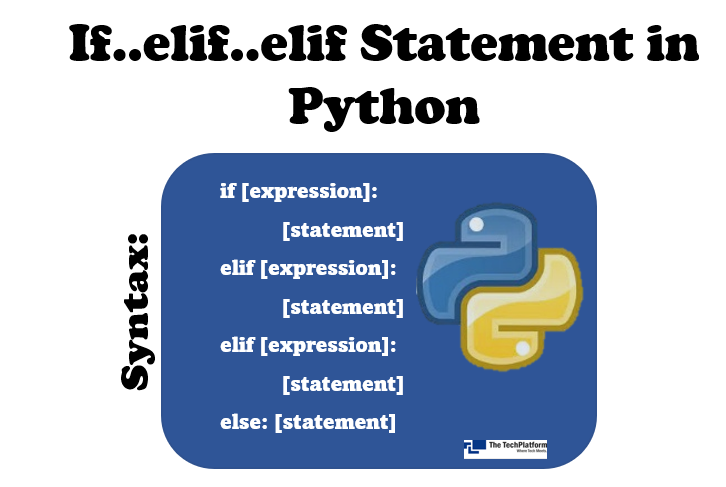top of page
Search

# If/elseif/elseif Statement in PythonThe elif statement adds another "decision" branch to if-else. In this article, we will learn how we can control the flow of execution using if-else statements.

#### Flowchart:## if..elif Condition in Python

This condition is used to include multiple conditional expressions after the if condition or between the if and else conditions.

Syntax:

```if [expression]:
[statement]
elif [expression]:
[statement]
elif [expression]:
[statement]
.
.
.
.
else:
[statement]```

When the if statement is false, the next elif expression is checked. When any one expression is true, the control goes outside the if-else block.

In case else is not specified, and all the statements are false, none of the blocks would be executed.

Example 1:

```price = 50

if price > 100:
print("Price is greater than 100")
elif price == 100:
print("Price is 100")
else :
print("Price is less than 100")```

Output:Example 2:

```inp_num = input("Enter a number: ")
40

inp_num = int(inp_num)

if inp_num == 0:
print(inp_num, "is Even")
elif inp_num%2==0:
print(inp_num, "is Even")
else:
print(inp_num, "is Odd")```

Output:## Nested If, elif, else condition

Python supports nested if, elif, and else condition. The inner condition must be with increased indentation than the outer condition, and all the statements under the one block should be with the same indentation.

Example:

```price = 100
quantity = 10
amount = price*quantity

if amount > 200:
if amount > 500:
print("Amount is greater than 500")
else:
if amount < 500 and amount > 400:
print("Amount is")
elif amount < 500 and amount > 300:
print("Amount is between 300 and 500")
else:
print("Amount is between 200 and 500")
elif amount == 200:
print("Amount is 100")
else:
print("Amount is less than 100")```

Output:The Tech Platform